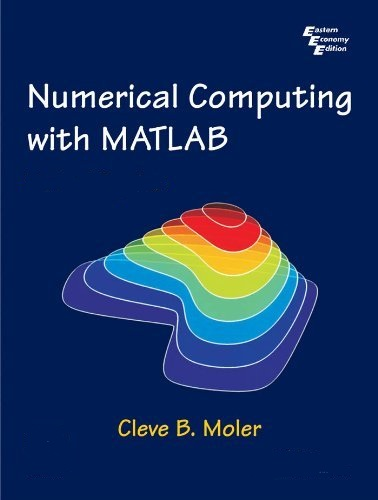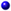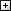Processing ......FreeComputerBooks.com Free Computer, Mathematics, Technical Books and Lecture Notes, etc.

Numerical Computing with MATLAB, Electronic (Web) Edition
How many runways in a particular airport? Click here to find out.
• Title Numerical Computing with MATLAB, Electronic (Web) Edition
• Author(s) Cleve B. Moler
• Publisher: Priniting: SIAM (July 25, 2008); eBook (Updated on September 16, 2013)
• License: Reproduction of single copies of this Web edition is permitted for individual use.
• Paperback 348 pages
• Language: English
• ISBN-10: 0898716608
• ISBN-13: 978-0898716603Book Description

Numerical Computing with MATLAB is a lively textbook for an introductory course in numerical methods, MATLAB, and technical computing. The emphasis is on the informed use of mathematical software; in particular, the presentation helps readers learn enough about the mathematical functions in MATLAB to use them correctly, appreciate their limitations, and modify them appropriately.

• The book makes extensive use of computer graphics, including interactive graphical expositions of numerical algorithms. It provides more than 70 M-files, which can be downloaded from the Web. Many of the more than 200 exercises involve modifying and extending these programs.
• The topics covered include an introduction to MATLAB; linear equations; interpolation; zeros and roots; least squares; quadrature; ordinary differential equations; Fourier analysis; random numbers; eigenvalues and singular values; and partial differential equations. Motivating applications include modern problems from cryptography, touch-tone dialing, Google page-ranking, atmospheric science, and image processing, as well as classical problems from physics and engineering.

This book is designed for a one-quarter or one-semester course primarily for students in science and engineering, at the advanced undergraduate or beginning graduate level. Prerequisites are calculus, some familiarity with ordinary differential equations and matrices, and some computer programming

This Electronic (Web) Edition includes changes and corrections made since the original edition was published in 2004.All CategoriesRecent BooksIT Research LibraryMiscellaneous BooksComputer LanguagesComputer ScienceData Science/DatabasesElectronic EngineeringJava and Java EE (J2EE)Linux and UnixMathematicsMicrosoft and .NETMobile ComputingNetworking and CommunicationsSoftware EngineeringSpecial TopicsWeb Programming